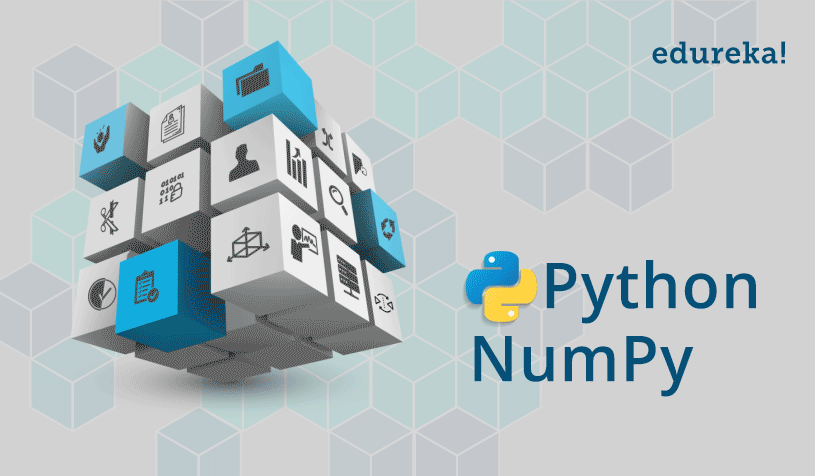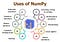# A-Z with Python Numpy Library.

The most fundamental module for scientific computing with Python Language. 🐍Credits: https://ssiddique.info/

Hola Readers, As we all are well aware of the fact that Python Is the most powerful language right now, in fact, Python has become the language that every Tech guy should know.

So this article is on one of the Python most important and most used libraries, i.e. NUMPY LIBRARY. Before starting If you want to know the basics of Python Programming you can see another article based entirely on the basics of Python Language. Click Here.

Starting with the Quote:

“Python is a great first language”, elicited, “Python is a great last language!”

- Noah Spurrier

# ✨ INTRODUCTION TO NUMPY.

## What is Numpy?

NumPy stands for ‘Numerical Python.’ It is a basic scientific library used in statistical functions, linear algebra, arithmetic operations, bitwise operations, etc.

It is basically the core library for scientific computing, which contains a powerful n-dimensional array object.Credits: https://www.edureka.co

We perform all the operations on the array elements. We can initialize these arrays in several ways.

The two basic Prerequisites for NumPy are Python and Mathematics. We need to know the Python basics to work with the NumPy module.

## Why NumPy arrays?

A numpy array is a powerful N-dimensional array object which is in the form of rows and columns.

Numpy can be put to use for storing, manipulation, and deletion of array elements. We can use it for sorting, indexing, and stacking of the array elements.

## Limitations of NumPy?

It was designed to address the problem of missing values. NumPy itself supports “nan” but the lack of cross-platform support within Python makes it difficult for the user. That’s why we may face problems when comparing values within the Python interpreter.

## Installation?

To install NumPy on Linux using pip, run the following command on the terminal:

`sudo pip3 install numpy`

To install NumPy on Windows using pop, run the following command on the command prompt. Make sure you have installed pip before you run the command to install NumPy.

`pip3 install numpy`

Then go to the IDE and use the import command import NumPy as np. 🤗

## Use of Numpy?Credits: https://techvidvan.com

# NumPy ndarray Objects.

## Array indexing and slicing:

Single-dimensional Numpy Array:

`import numpy as npa = np.array([1,2,3])print(a)O/P — [1 2 3]`

Multi-dimensional Array:

`a=np.array([(1,2,3),(4,5,6)])print(a)O/P — [[ 1 2 3][4 5 6]]`

An `ndarray` is a (usually fixed-size) multidimensional container of items of the same type and size. The number of dimensions and items in an array is defined by its `shape`, which is a `tuple` of N non-negative integers that specify the sizes of each dimension. The type of items in the array is specified by a separate data-type object (dtype), one of which is associated with each ndarray.

The most important attributes of ndarray objects are:

• ndarray.ndim is the number of dimensions (more often called “axes”) of the array.
• ndarray.shape is the size of the array. It is a tuple of natural numbers showing the length of the array along each axis. For a matrix of n rows and m columns, the shape would be (n, m). The number of elements of the shape tuple is ndim.
• ndarray.size is the number of elements in the array which is n*m from the array shape.
• ndarray.dtype is an object describing the type of array elements. You can define dtype using standard Python data types. NumPy here provides a whole bunch of possibilities, both built-in, for example, bool_, character, int8, int16, int32, int64, float8, float16, float32, float64, complex64, object_, and the ability to define your own data types, including composite ones.
• ndarray.itemsize is the size of each element in the array in bytes.
• ndarray.data is a buffer containing the actual elements of the array. Usually, this attribute is not used much as the easiest way to access array elements is using indices.

Slicing is basically extracting a particular set of elements from an array. This slicing operation is pretty much similar to the one which is there in the list as well.

For Example:

`import numpy as npa=np.array([(8,9),(10,11),(12,13)])print(a[0:2,1])Output — [9 11]`

As you can see in the above code, only 9 and 11 gets printed. Now when I have written 0:2, this does not include the second index of the third row of an array. Therefore, only 9 and 11 gets printed else you will get all the elements i.e [9 11 13].

Python is the “most powerful language you can still read”.

- Paul Dubois

## Memory layout of ndarray:

An instance of class `ndarray` consists of a contiguous one-dimensional segment of computer memory (owned by the array, or by some other object), combined with an indexing scheme that maps N integers into the location of an item in the block. The ranges in which the indices can vary is specified by the `shape` of the array.

NumPy is flexible, and `ndarray` objects can accommodate any strided indexing scheme.

Views and copies:

The main difference between a copy and a view of an array is that the copy is a new array, and the view is just a view of the original array.

`The copy returns None.The view returns the original array.`

Example of Copy:

`import numpy as nparr = np.array([1, 2, 3, 4, 5])x = arr.view()arr = 42print(arr)print(x)`

The copy SHOULD NOT be affected by the changes made to the original array.

Example of View:

`import numpy as nparr = np.array([1, 2, 3, 4, 5])x = arr.view()arr = 42print(arr)print(x)`

The view SHOULD be affected by the changes made to the original array.

## Creating arrays:

One of the simpler ones is to create an array from regular Python lists or tuples using the numpy.array() function:

`import numpynumpy.array([1,2])# result: array([1, 2])`

To create multidimensional arrays in NumPy, just use the multidimensional list in the array function.

`import numpynumpy.array([[1,2],[3,4]])# result: array([[1, 2],# [3, 4]])`

If you want to create an array and initialize the content to 0, you can use zeros() function and if you want to initialize the array content to 1 use ones() function, eye () function to create an identity matrix, empty() function to create empty array with random numbers, arange() to create a sequence of numbers.

## Array data type:

By default Python have these data types:

• `strings`
• `integer`
• `float`
• `boolean`
• `complex`

NumPy has some extra data types, and refer to data types with one character, like `i` for integers, `u` for unsigned integers, e.t.c.

• `i` - integer
• `b` - boolean
• `u` - unsigned integer
• `f` - float
• `c` - complex float
• `m` - timedelta
• `M` - DateTime
• `O` - object
• `S` - string
• `U` - Unicode string
• `V` - void

Check Datatype using the following code:

`import numpyp = numpy.arange(20,50, 5)p.dtype# result: dtype(‘int64’)`

# Linear Algebra with NumPy.

The Linear Algebra module of NumPy offers various methods to apply linear algebra on any NumPy array.

The NumPy `ndarray` class is used to represent both matrices and vectors. To construct a matrix in NumPy we list the rows of the matrix in a list and pass that list to the NumPy array constructor.

The SciPy library also contains a `linalg` submodule and there is overlap in the functionality provided by the SciPy and NumPy submodules.

# Using NumPy Arrays.

## Vectorized operations:

Since Numpy arrays is an array that contains data of a single type only, so there is a concept of vectorized operations on NumPy that allows the use of more optimal and pre-compiled functions and mathematical operations on NumPy array objects and data sequences.

For example:

Using the vectorized sum method on NumPy array.

`# importing the modulesimport numpy as npimport timeit# vectorized sumprint(np.sum(np.arange(15000)))print("Time taken by vectorized sum : ", end = "")%timeit np.sum(np.arange(15000))`

## Universal functions:

A universal function (or ufunc for short) is a function that operates on `ndarrays` in an element-by-element fashion, supporting array broadcasting, type casting, and several other standard features. That is, a ufunc is a “vectorized” wrapper for a function that takes a fixed number of specific inputs and produces a fixed number of specific outputs.

Some of the basic universal functions in Numpy are:

Trigonometric functions: It includes functions like- sin, cos, tan, arcsin, arccos, arctan, etc.

Statistical functions: It includes functions like- mean, median, variance, minimum, etc.

Bit-twiddling functions: It includes functions like- bitwise_and, bitwies_or, bitwise_xor, etc.

For example:

## Broadcasting :

The Broadcasting refers to the ability of NumPy to treat arrays of different shapes during arithmetic operations.

`import numpy as np a = np.array([1,2,3,4]) b = np.array([10,20,30,40]) c = a * b print cO/P: [10   40   90   160]`

The smaller array is broadcast to the size of the larger array so that they have compatible shapes.

## Shape manipulation/Array manipulation:

There is reshape() function that allows us to give a new shape to an array without changing its data.

This will be a new view object as a return value or it will be a copy.

`import numpy as np x = np.array([[2,3,4], [5,6,7]]) np.reshape(x, (3, 2)) O/P: array([[2, 3], [4, 5], [6, 7]])`

Bonus: One can even check the Dates of yesterday, today, and tomorrow using the Numpy;

Thanks For Reading. 😇

## Nerd For Tech

From Confusion to Clarification

## Nerd For Tech

NFT is an Educational Media House. Our mission is to bring the invaluable knowledge and experiences of experts from all over the world to the novice. To know more about us, visit https://www.nerdfortech.org/. Don’t forget to check out Ask-NFT, a mentorship ecosystem we’ve started

Written by

## Amandeep

“Technical content writer”## Nerd For Tech

NFT is an Educational Media House. Our mission is to bring the invaluable knowledge and experiences of experts from all over the world to the novice. To know more about us, visit https://www.nerdfortech.org/. Don’t forget to check out Ask-NFT, a mentorship ecosystem we’ve started

## More From Medium

Medium is an open platform where 170 million readers come to find insightful and dynamic thinking. Here, expert and undiscovered voices alike dive into the heart of any topic and bring new ideas to the surface. Learn more

Follow the writers, publications, and topics that matter to you, and you’ll see them on your homepage and in your inbox. Explore

If you have a story to tell, knowledge to share, or a perspective to offer — welcome home. It’s easy and free to post your thinking on any topic. Write on Medium